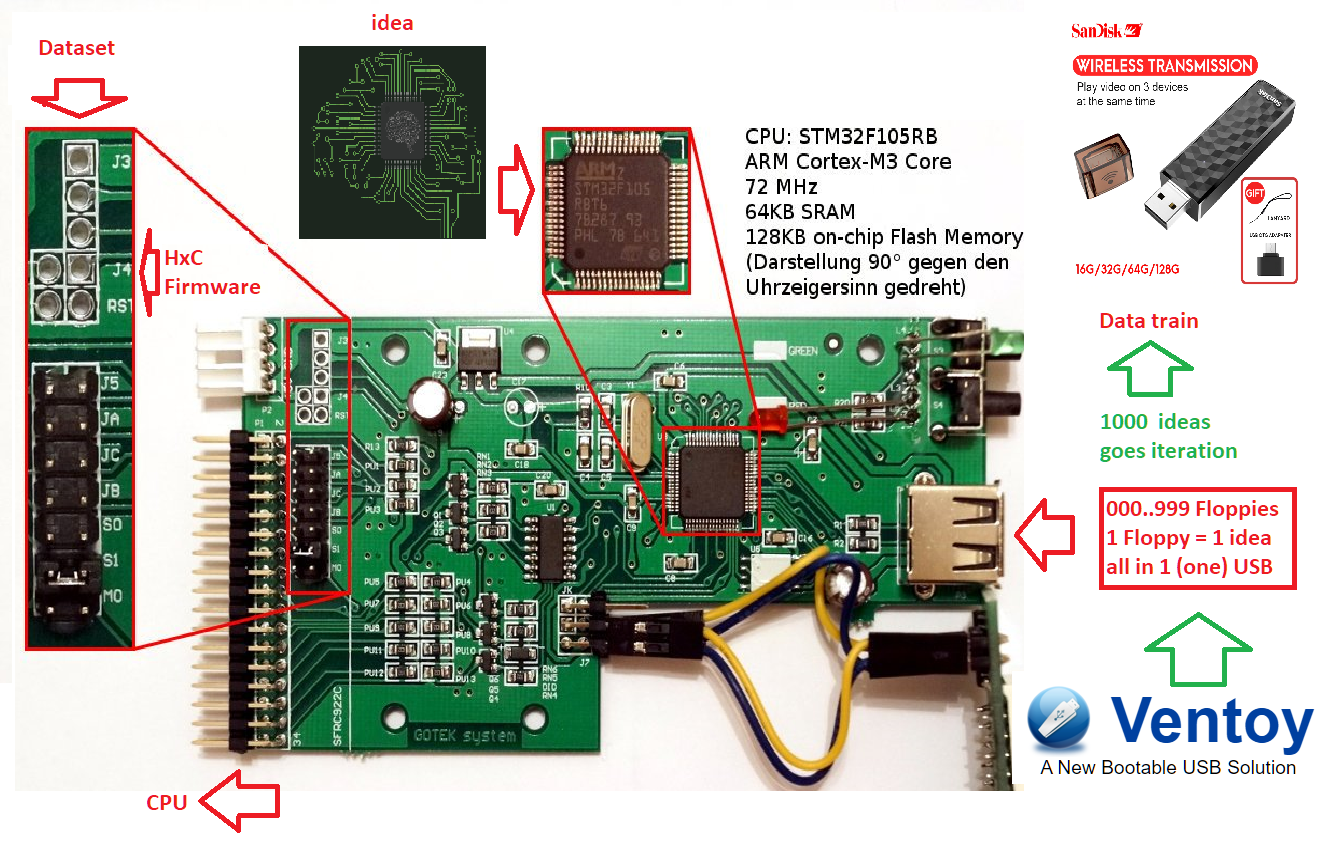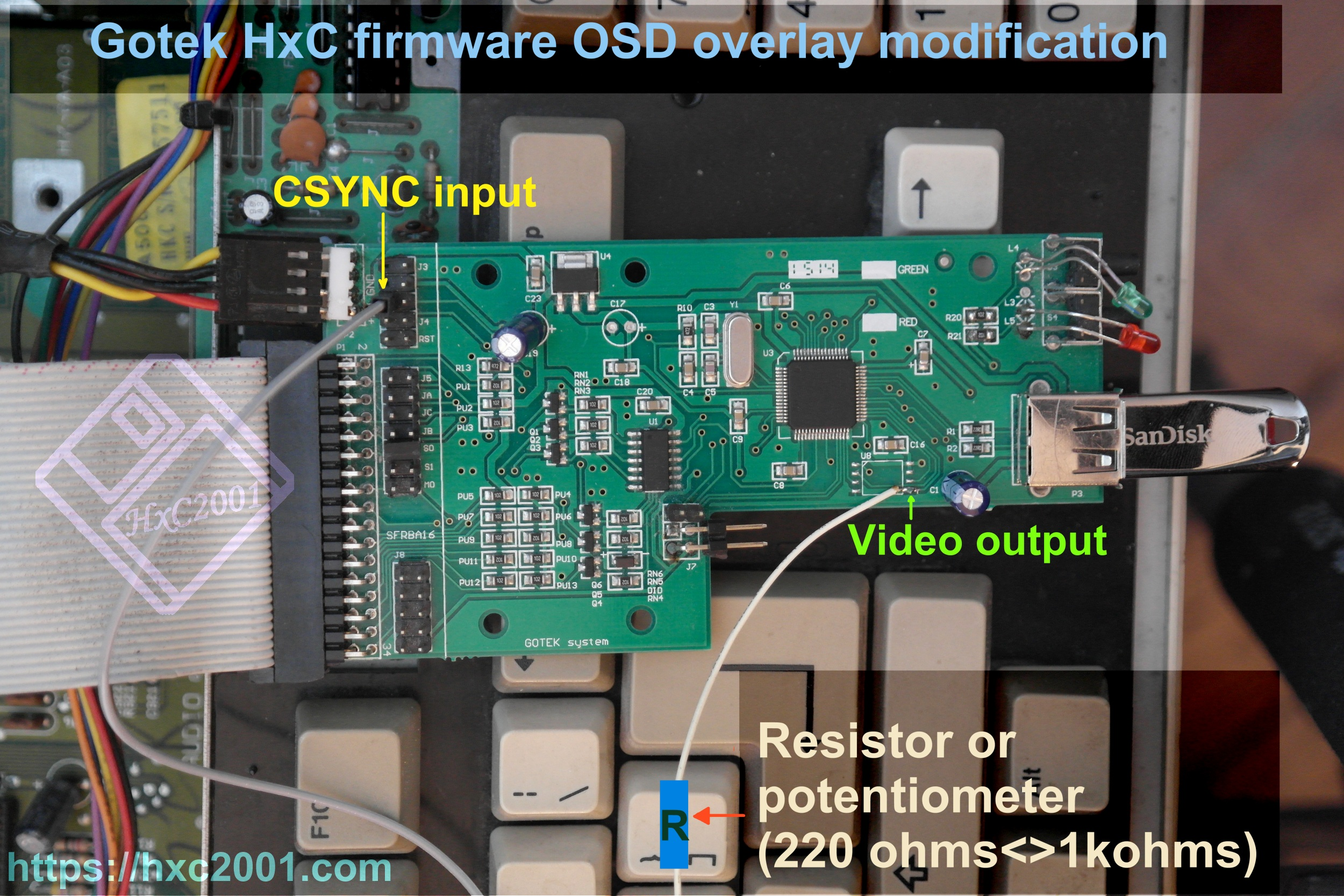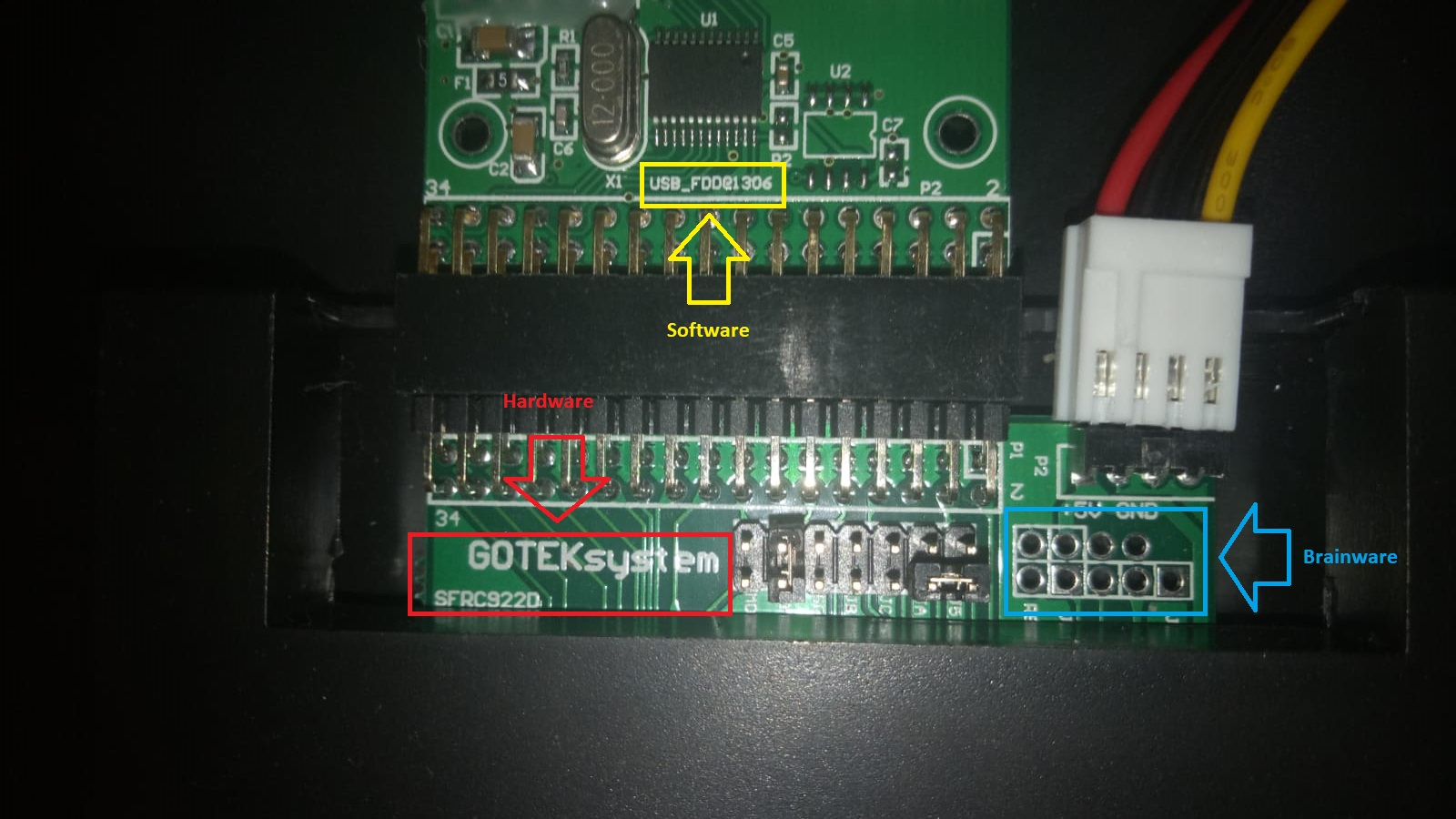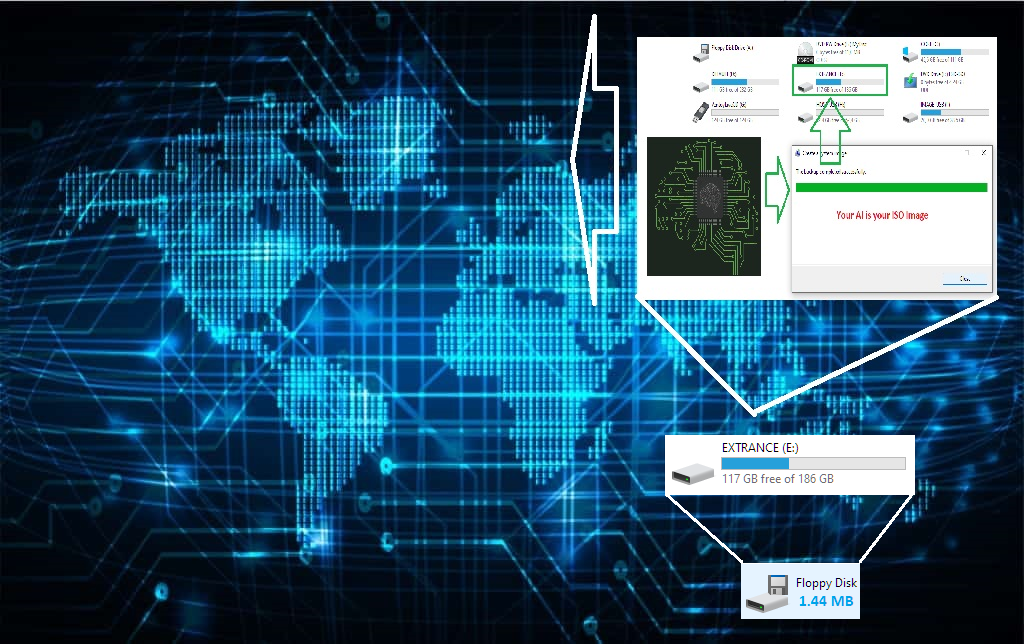# Replication Fork

``````---+-----+-----
1 |  1  |  4
---+-----+-----
2 |  5  | 14
---+-----+-----
3 | 15  | 26
---+-----+-----
4 | 27  | 40
---+-----+-----
5 | 41  |{47}
---+-----+-----
6 | 48  | 54
---+-----+-----
7 | 55  |{71}
---+-----+-----
8 | 72  | 75
---+-----+-----
``````
``````---+-----+-----
1 | 1   | 5    🡰--19--
---+-----+-----        |
2 | 6   | 8           |
---+-----+-----        |
3 | 9   | 26    --289-¤-exponentiation zone
---+-----+-----        |
4 | 27  | 28          |
---+-----+-----        |
5 | 29  | 29   ➤--18--
---+-----+-----
6 | 30  | 31   🡰--18--
---+-----+-----        |
7 | 32  | 44          |
---+-----+-----        |
8 | 45  | 46    --329-¤-multiplication zone
---+-----+-----        |
9 | 47  | 49          |
---+-----+-----        |
10 | 50  | 50   ➤--17--
---+-----+-----
11 | 51  | 53   🡰--17--
---+-----+-----        |
12 | 54  | 59          |
---+-----+-----        |
13 | 60  | 82    --168-¤-addition zone
---+-----+-----        |
14 | 83  |{102}        |
---+-----+-----        |
15 |{103}| 110  ➤--16--
---+-----+-----
``````

20 x 10 = 200 = 16 x 6 + (10² + 14 - 10) = 96 + 114 - 10 = 96 + 104 = 48 + 48 + 104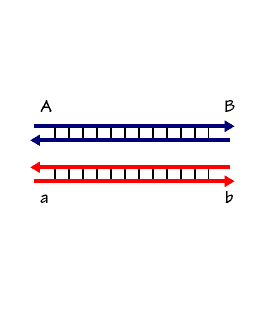!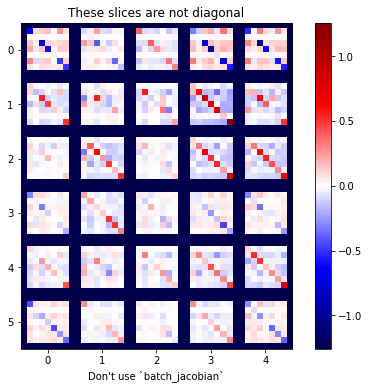Let's see how triangular numbers come into the picture. A triangular number is a number that can be represented by a pattern of dots arranged in an equilateral triangle with the same number of dots on each side at equal distance from each other.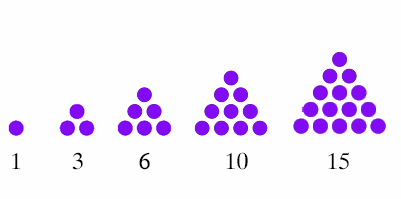``````                largest part=21 → 11+13+12=36 →  MEC30
↓                      |
---+-----+-----+-----+-----+                   ↓
1 | 19  | 1   | 20  | 21  |-------------------|-----
---+-----+-----+-----+-----+                   ↓     |
2 | 18  | 21  | 39  | 60  |-------------------      |
---+-----+-----+-----+-----+                   |     |
3 |{63} | 40  | 103 | 143 |-------------      |     |
---+-----+-----+-----+-----+             |     |     |
4 | 37  | 104 | 141 | 245 |-------      |     |     |
---+-----+-----+-----+-----+       |     |     |     |
5 | 10* | 142 | 152 | 294 |- 11** | 13  | 12  | 12  | 18
---+-----+-----+-----+-----+       |     |     |     |
6 | 24  | 153 | 177 | 332 |-------      |     |     |
---+-----+-----+-----+-----+             |     |     |
7 | 75  | 178 | 253 | 431 |-------------      |     |
---+-----+-----+-----+-----+                   |     |
8 | 30  | 254 | 284 | 538 |-------------------      |
---+-----+-----+-----+-----+                   ↓     |
9 | 1   | 285 | 286 | 571 |-------------------|-----
===+=====+=====+=====+=====+                   ↓
45 | 277 |                      ← 11+13+12=36 ←  MEC30
---+-----+                                     |
↑
Note:
10* stands as the central rank
11** stands as the central parts
``````

## Windows registry

This software uses Windows Script Host to read and write to the registry. Read, Write, List and do all sorts of funky stuff to the windows registry using node.js and windows script host.``````7 x 11 = 77 = 99 - 22 = 11 x (9 -2)

layer | node | sub |  i  |  f
------+------+-----+----------
|      |     |  1  | -----------------------  71 = 72-1
|      |  1  +-----+                        |
|  1   |     |  2  | (5)                    |
|      |-----+-----+                        |
|      |     |  3  | ---------              |
1   +------+  2  +-----+----      |             |
|      |     |  4  |          5x ---        |
|      +-----+-----+          |     |       |
|  2   |     |  5  | (7) -----      |       |
|      |  3  +-----+                |       |
289+11=300   |     |  6  |                |       |
------+------+-----+-----+----- 72 x 6    7x --- 11x = 77
|      |     |  7  |                |       |
|      |  4  +-----+                |       |
|  3   |     |  8  | (11)  ---      |       |
|      +-----+-----+          |     |       |
|      |     |  9  |          2x ---        |
2   +------|  5  +-----+-----     |             |
|      |     |  10 | ---------              |
|      |-----+-----+                        |
|  4   |     |  11 | (13) ------------------  71 = 72-1
|      |  6  +-----+
329+71=400   |     |  12 |------------------------  70 = 72-2
------+------+-----+-----+
|      |     |  13 |
|      |  7  +-----+
|  5   |     |  14 | (17)
|      |-----+-----+
|      |     |  15 |
3   +------+  8  +-----+----- 42 x 6
|      |     |  16 |
|      |-----+-----+
|  6   |     |  17 | (19)
|      |  9  +-----+
168+32=200   |  |  |  18 |------------------------  68 = 72-4
------|------|--|--+-----+
900 -----
``````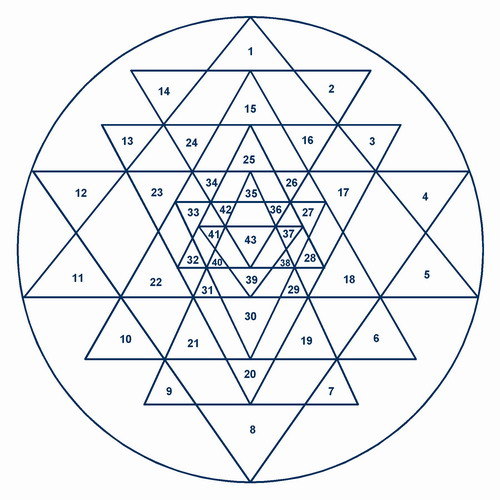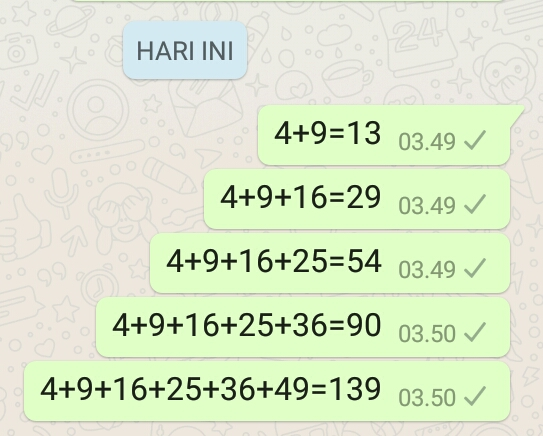### Prime templates

In the windows registry a key may have a default value. When enumarting value names, the default value's name will be empty.

The number 28 depicted as 28 balls arranged in a triangular pattern with the number of layers of 7 ([Wikipedia).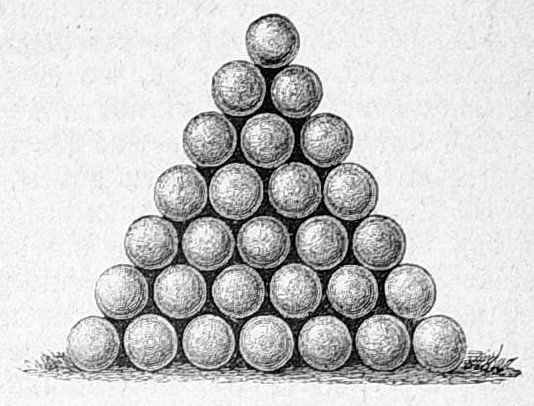This presents a minor problem when including the empty value in a set with other values since it cannot be safely named with anything but the empty string, for fear of collision with other values.


``````const regedit = require('regedit').promisified

async function main() {
const listResult = await regedit.list('HKCU\\SOFTWARE')
console.log(listResult)

await regedit.createKey(['HKLM\\SOFTWARE\\MyApp2', 'HKCU\\SOFTWARE\\MyApp'])
await regedit.putValue({
'HKCU\\SOFTWARE\\MyApp': {
Company: {
value: 'Moo corp',
type: 'REG_SZ'
}
},
'HKLM\\SOFTWARE\\MyApp2': {
test: {
value: '123',
type: 'REG_SZ'
}
}
})
}

main()
``````

Now the following results: Due to the convolution and starting from the desired value of the prime position pairs, the product templates and prime numbers templates of the prime number 7 lie in the numerical Double strand parallel opposite.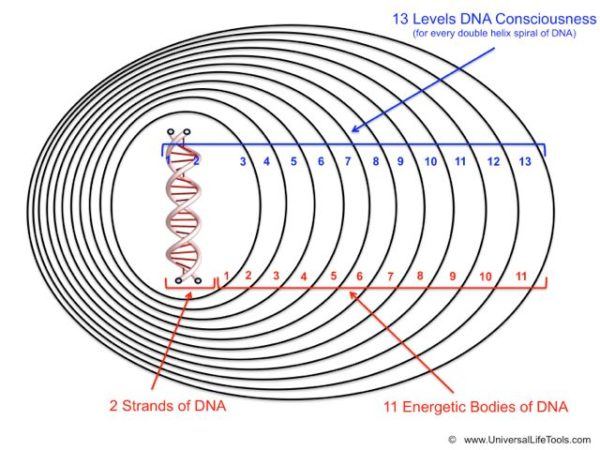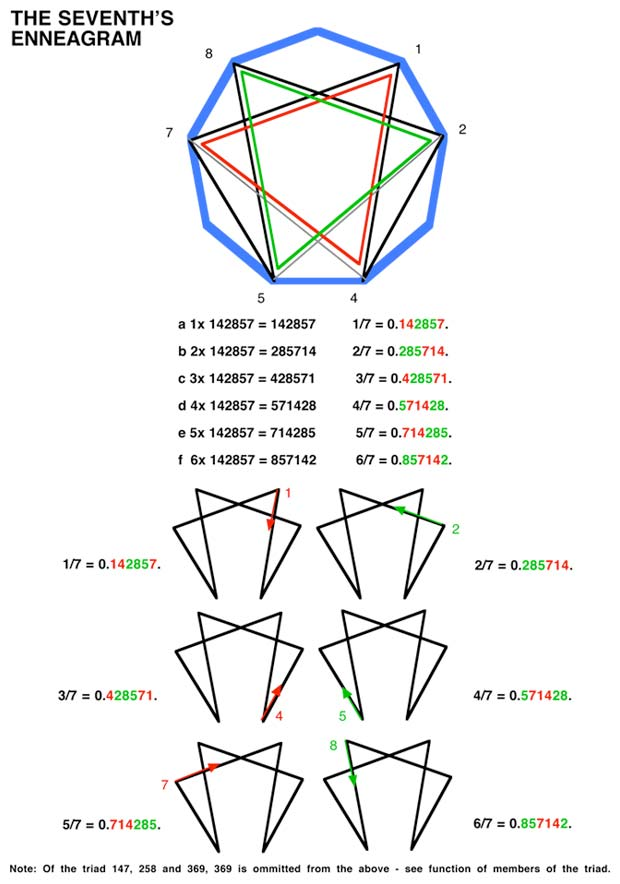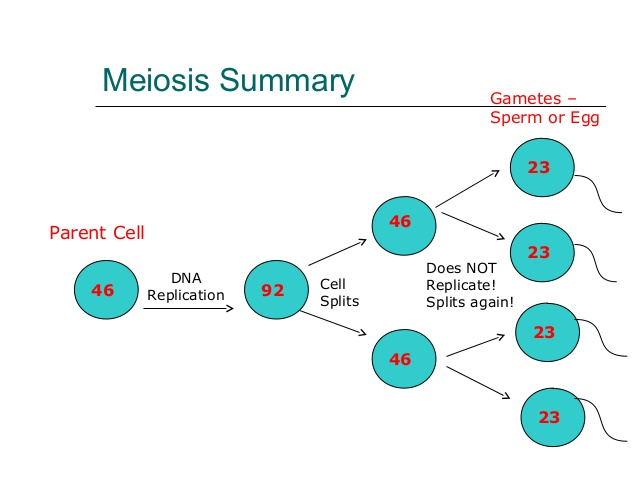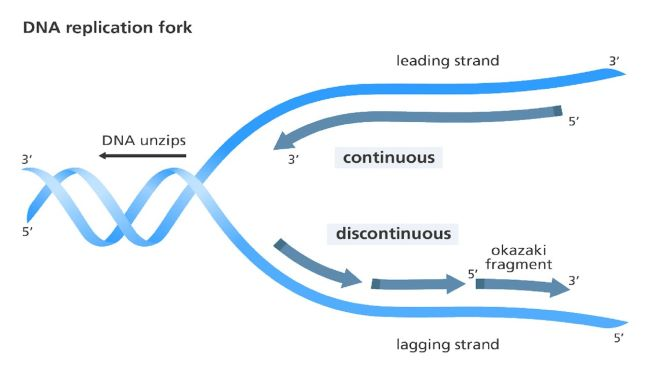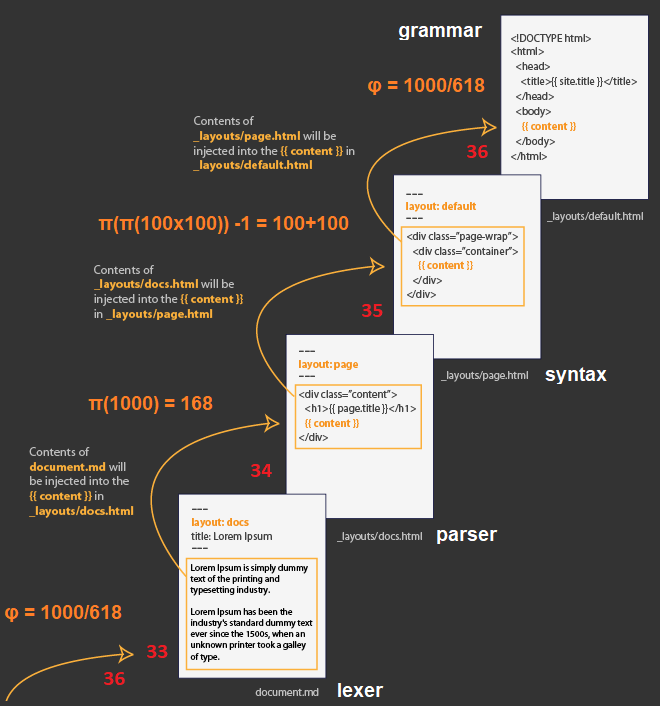### Anti paralled

The Workflow by this frameworks will absorbe the way of how a DNA is read DNA polymerase which is proceed in the 3′ to 5′ direction, while the new strand is synthesized in the 5' to 3' direction. This scheme is widely known as anti paralled direction.

Since the leading and lagging strand templates are oriented in opposite directions at the replication fork, a major issue is how to achieve synthesis of new lagging strand DNA, whose direction of synthesis is opposite to the direction of the growing replication fork (Wikipedia).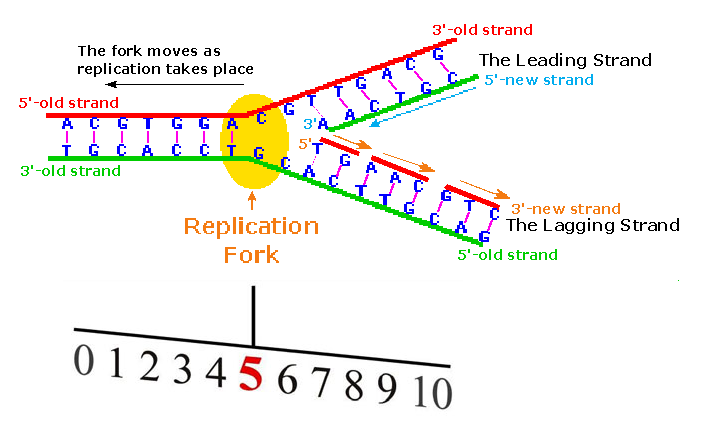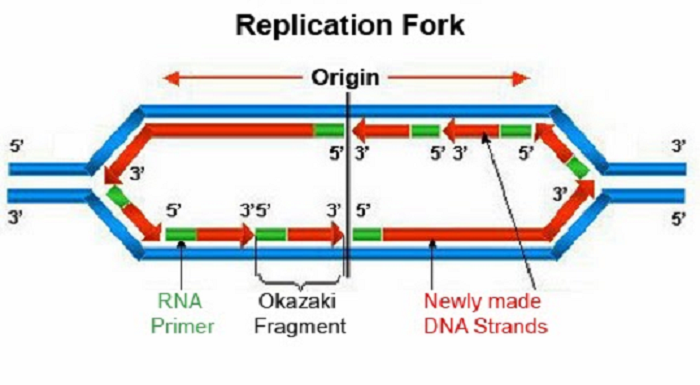By DNA System it will act as the Replication Fork that exchange along with their residual objects. You may see that it begin with two (2) prime identities of (2,3) and end up with the tensor of 100 to 50 conforms the ζ(s)=1/2.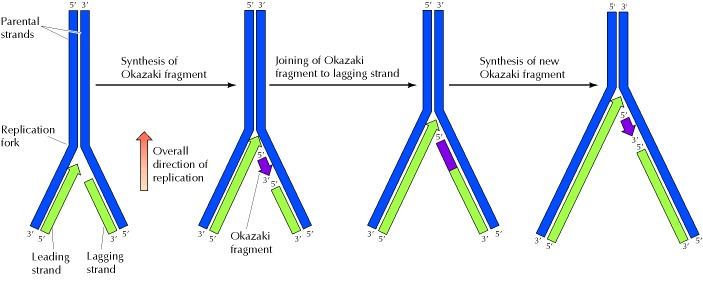286 - 4x2 = 278

``````329   |      |  6  +----------+-----+-----+-----+                                     |
|     |      |     |    19:12 |  10 |  60 | {70} (36) -------› Φ                      |
------+------+-----+----------+-----+-----+                                          ---
|      |     |    20:13 |  90 |  90 (38) ‹-------------- ¤                      |
|      |  7  +----------+-----+                                                 |
|  5   |     |       14 |            -----------------------------             17¨  class
|      |-----+----------+           |                             |             |
|      |     |       15 |           |       LEADING SCHEME        |             |
3   +------+  8  +----------+-----      |    (Multiplication Zone)    |            ---
|      |     |       16 |           |                             |             |
|      |-----+----------+-----+      -----------------------------              |
|  6   |     |    28:17 | 100 |                                                19¨  object
168   |      |  9  +----------+-----+                                                 |
|     |      |     |    29:18 | 50  | 50(68) ---------> Δ18                           |
------|------|-----+----------+-----+                                                ---
``````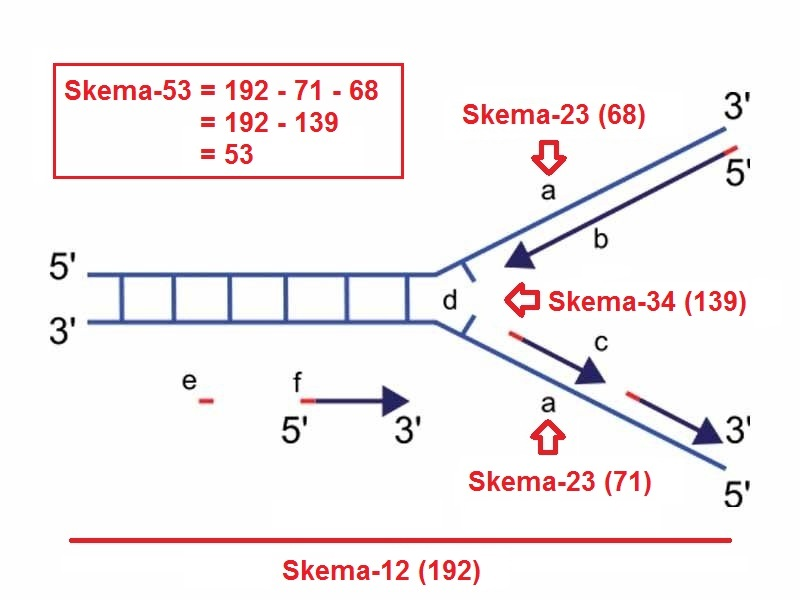``````layer | node | sub |    i     |   f
------+------+-----+----------+-----+-----+-----+                                    ---
|      |     |    1,2:1 |   1 |  30 |  40 | 71 (2,3) ‹-------------------       |
|      |  1  +----------+-----+-----+-----+                              |      |
|  1   |     |        2 |                                                |      5¨  encapsulation
|      |-----+----------+            -----------------------------       |      |
|      |     |        3 |           |                             |      |      |
1   +------+  2  +----------+----       |       LAGGING SCHEME        |      |     ---
|      |     |        4 |           |    (Exponentiation Zone)    |      |      |
|      +-----+----------+           |                             |      |      |
|  2   |     |        5 |           ------------------------------       |      7¨  abstraction
289   |      |  3  +----------+                                                |      |
|     |      |     |        6 |  ‹---------------------------- Φ               | {6®} |
------+------+-----+----------+-----+-----                                     |     ---
``````

## Exponentiation Zone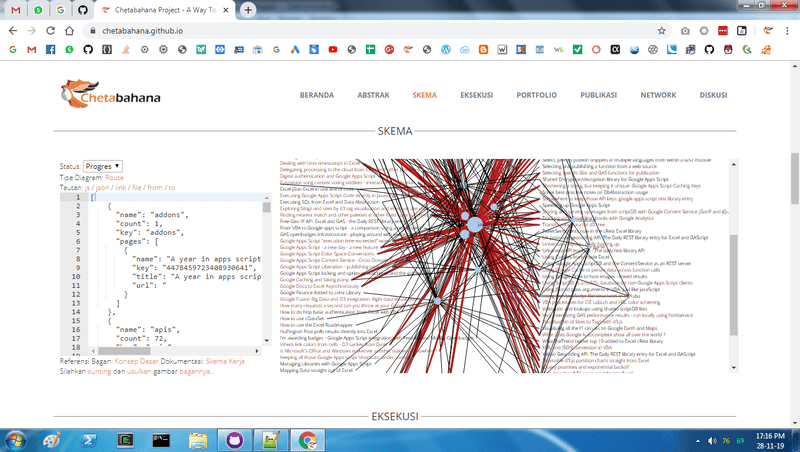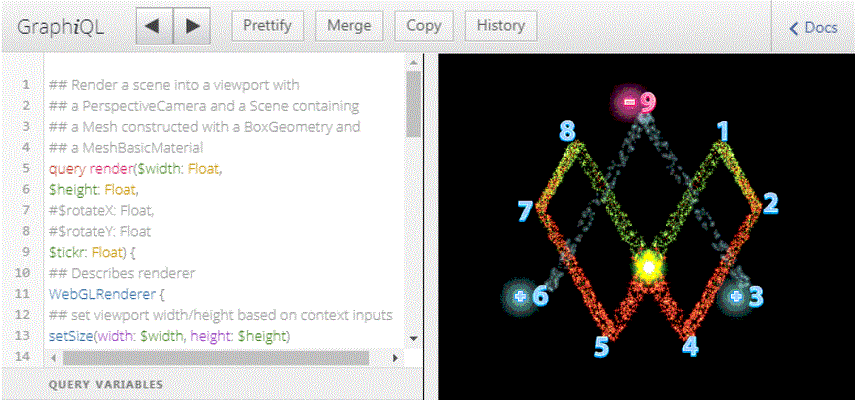By this flowchart we could deploy models on device and in the browser from image classification, text embeddings, audio, and video action recognition where you can browse trained models and datasets from across the machine learning ecosystem.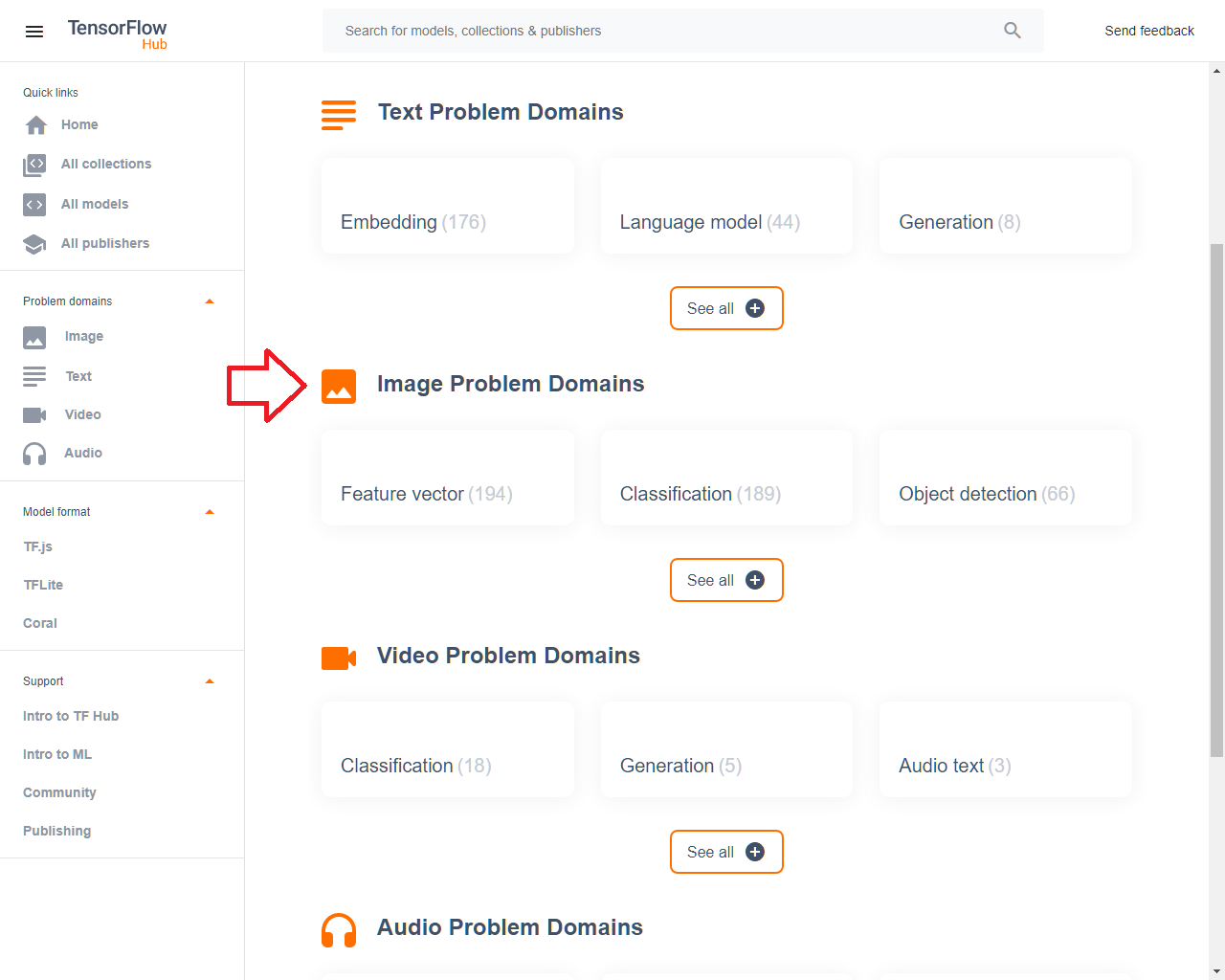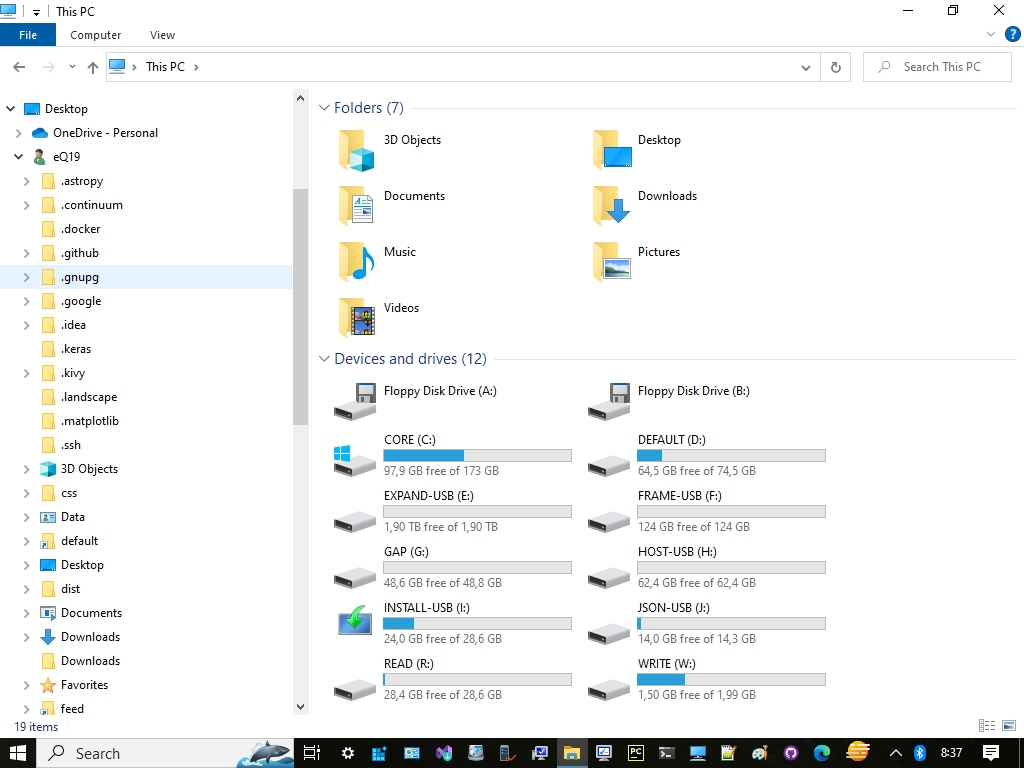### Multiplicatio Zone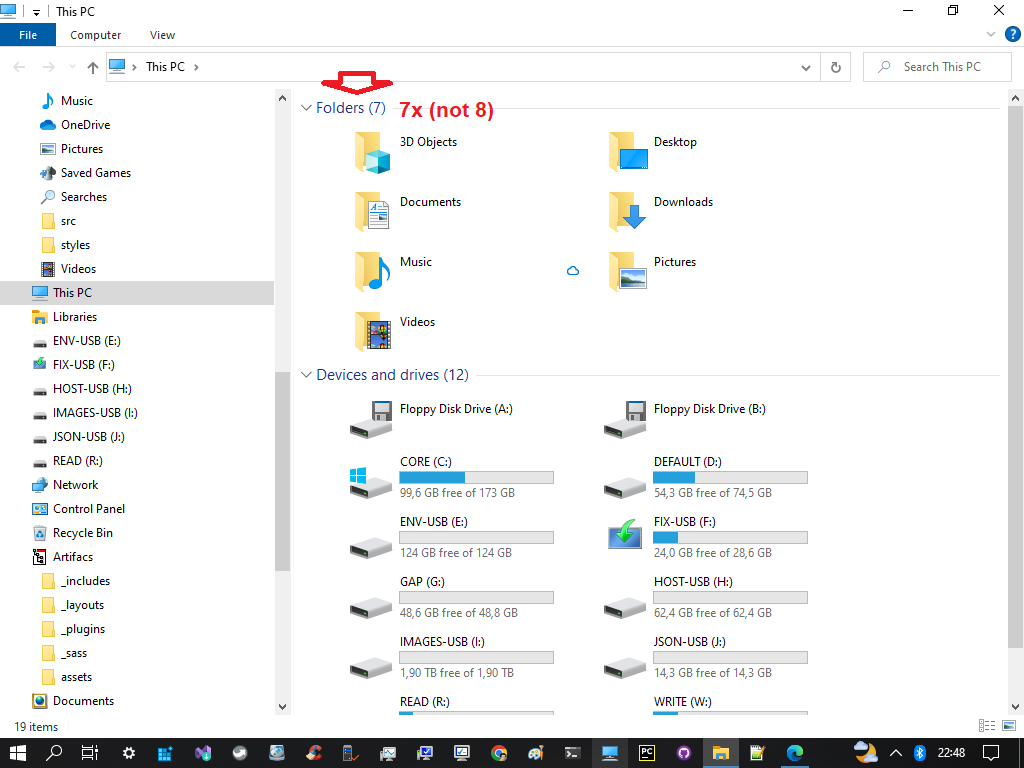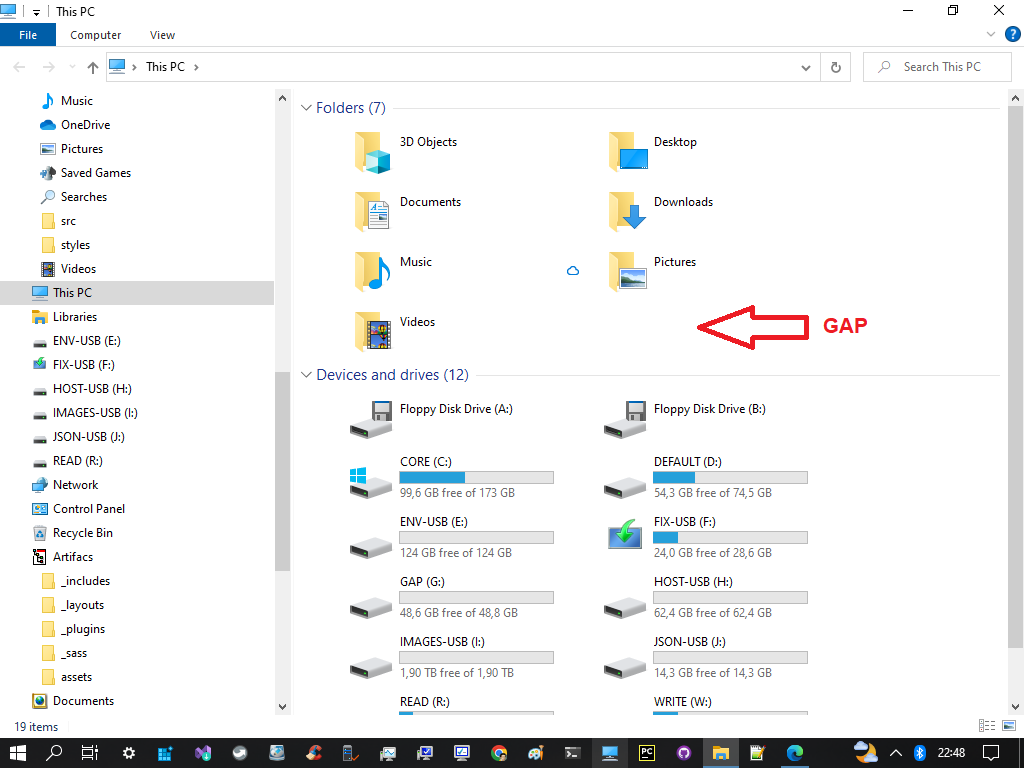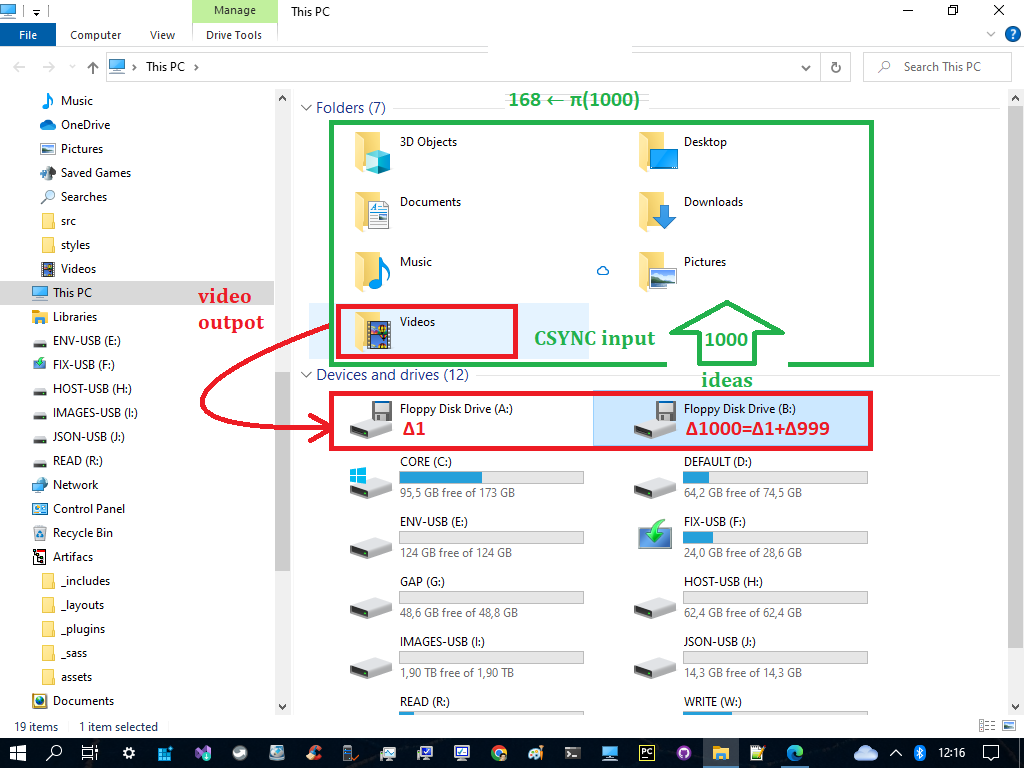7 X (7+6) X (7+12) = 7 X 13 X 19 = 7 X 247 = 1729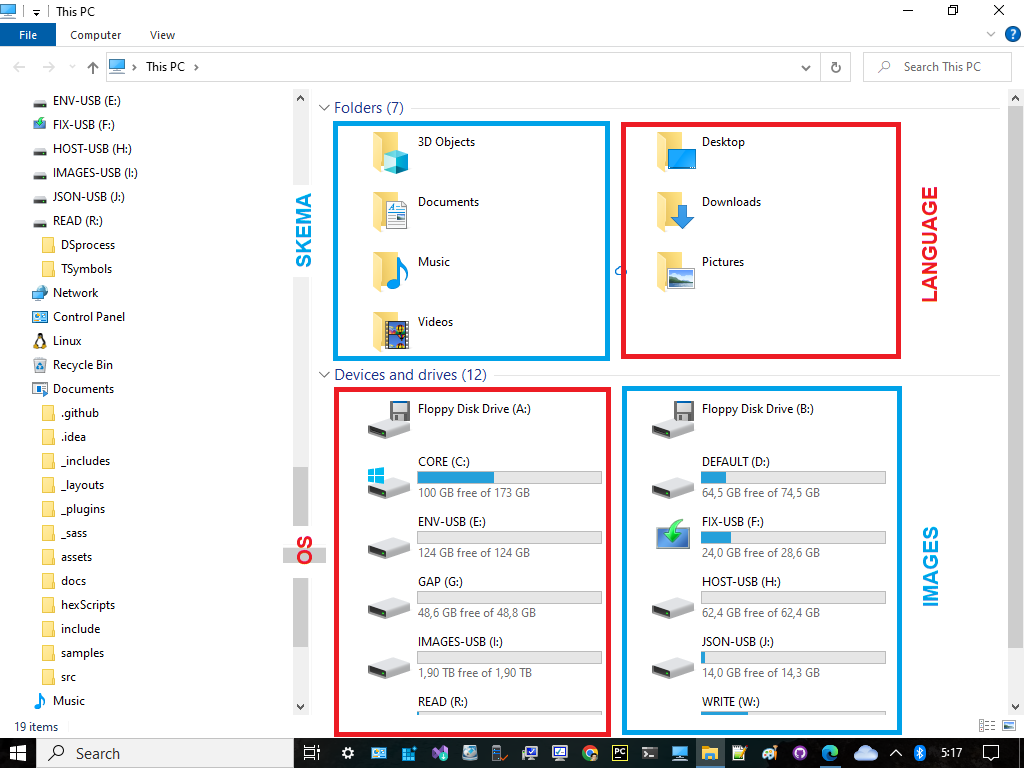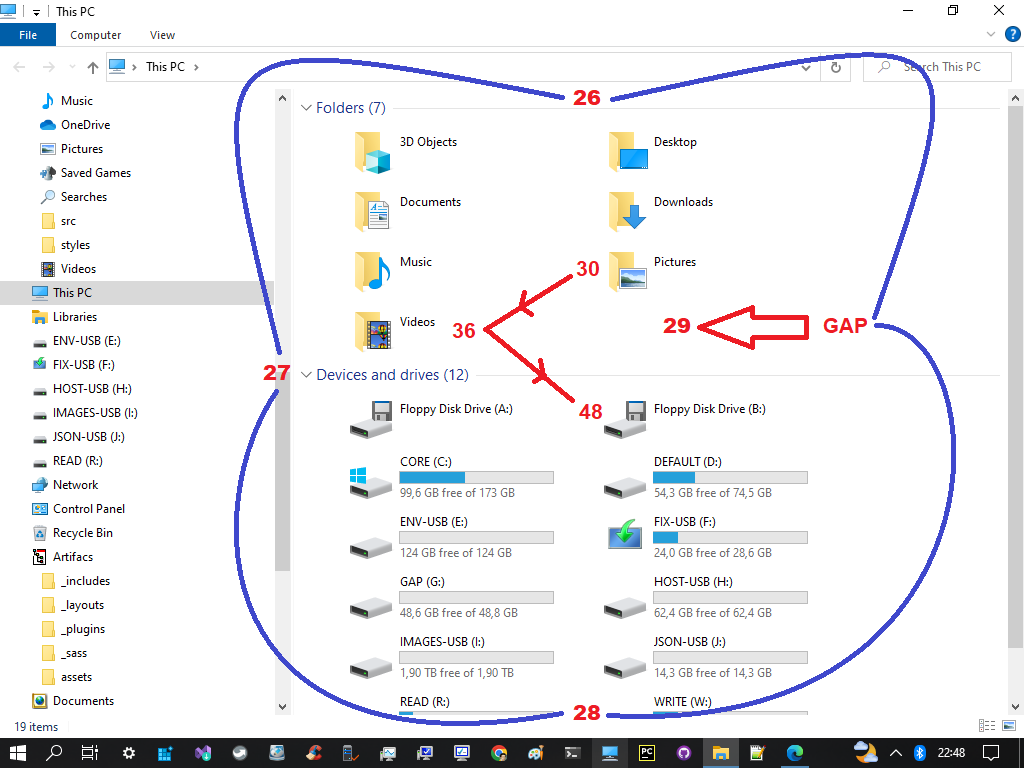By all of the explanation above we concluded that this recycling is started by the tensor of bilateral 9 sums originated from the power of number two (2) which is resulting the congruent property of number 18.This property would tend the ballancing scheme of MEC30 so it will let 30-18=12 pairing with another 12 of 24 spins prime hexagon. The 24 goes to the center of True Prime Pairs by the prime pair 13 and 11 and let the crancks of 2,3,5,7 inside the 10 ranks.

This is where Goldbach's conjecture, which was also known to Euler, helps. Goldbach considered the distribution of primes from the perspective of the line numbers that represent a separate set of numbers, as shown. So here also helps that the Riemann conjecture is essentially identical point, with the Goldbach conjecture.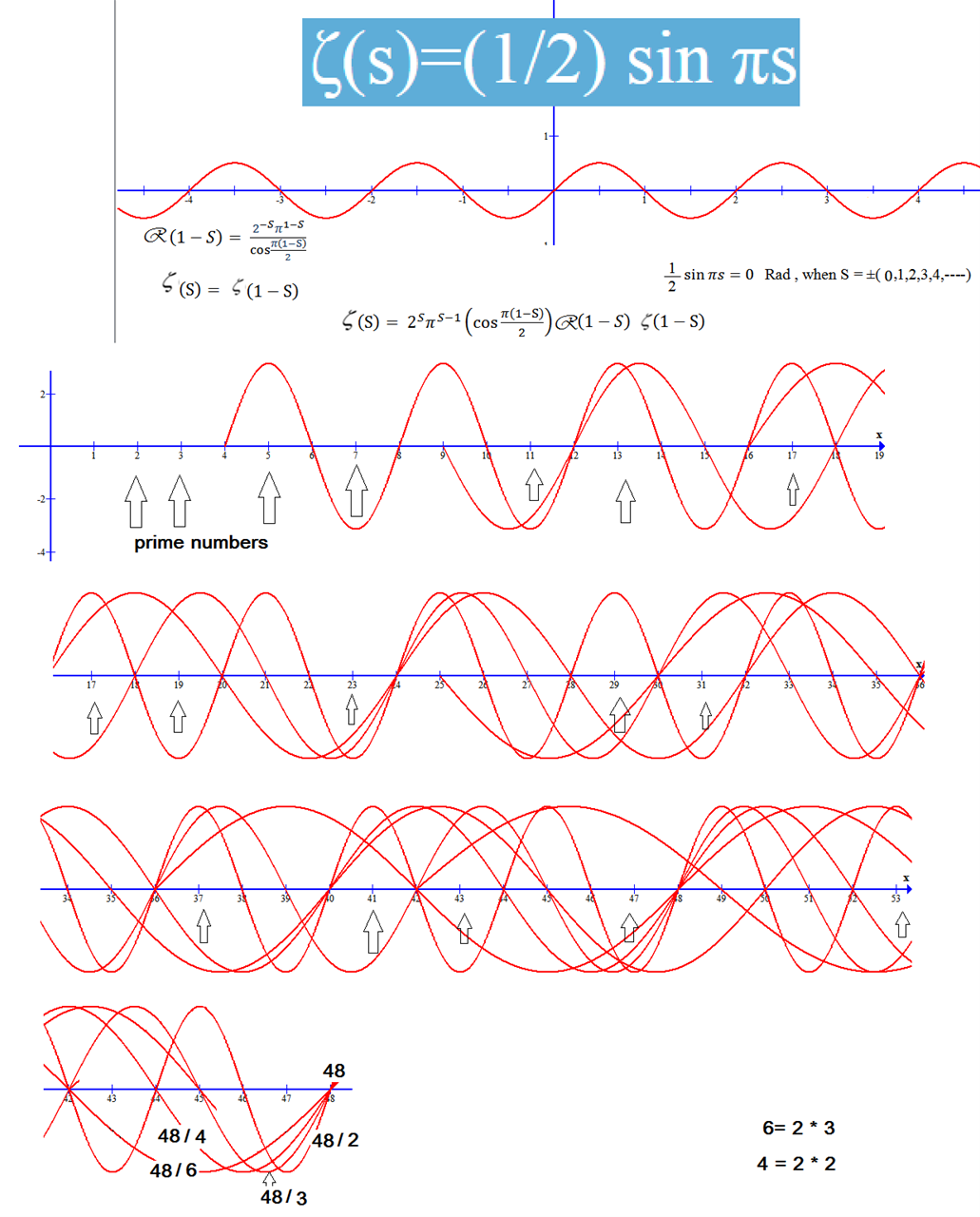The 10 ranks will coordinate with the 18 to raise up the symmetrical behaviour of 12+24=36 which is prime pair 17+19=36 and let the 2 and 3 out of 2,3,5,7 to begin a new cycle while the 5,7 will pair the 11,13 and 17,19 as True Prime Pairs.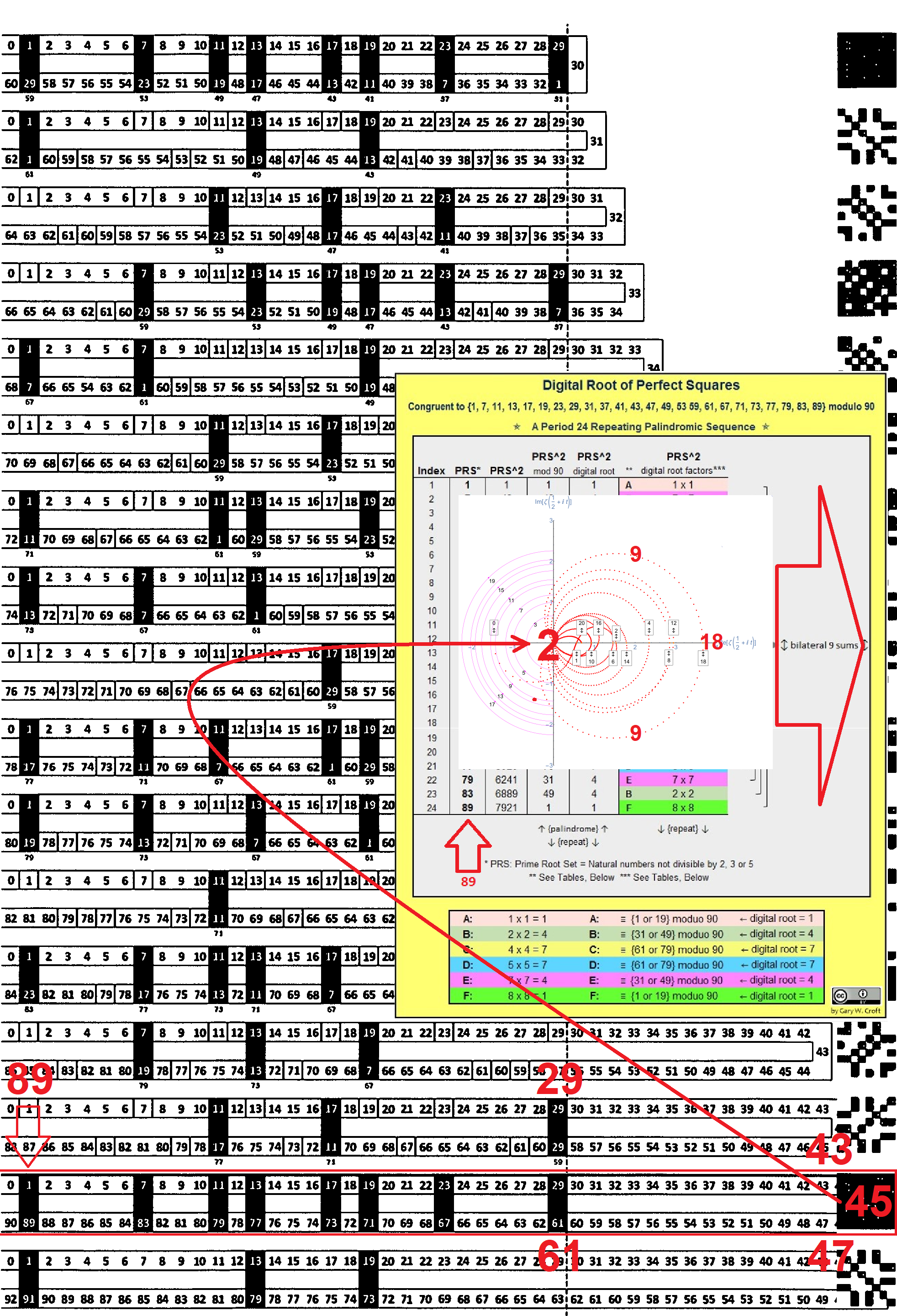With the above description then the instance configuration is via matrix integration from tensorflow which is placed at the end position to the turning point 100 vs 50 to the format (2,3) i.e. at id: 68.

π(1000) = π(Φ x 618) = 168 = 100 + 68 = (50x2) + (66+2) = 102 + 66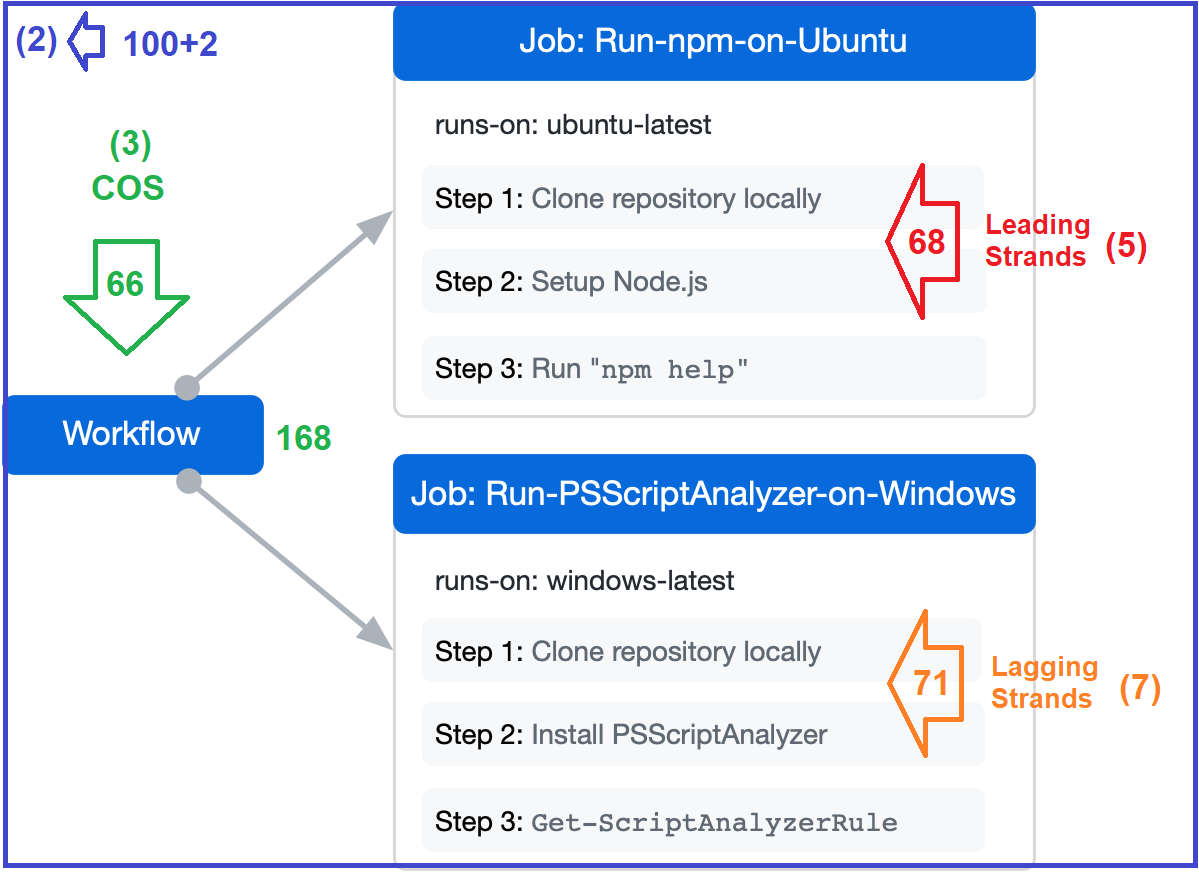### Identical point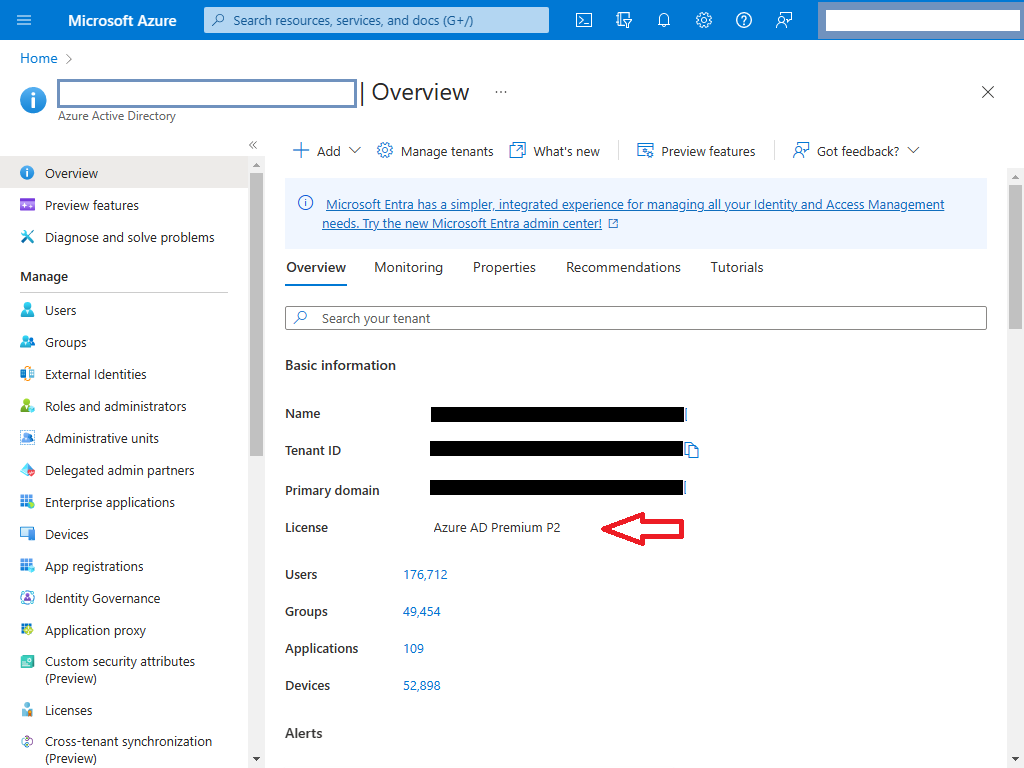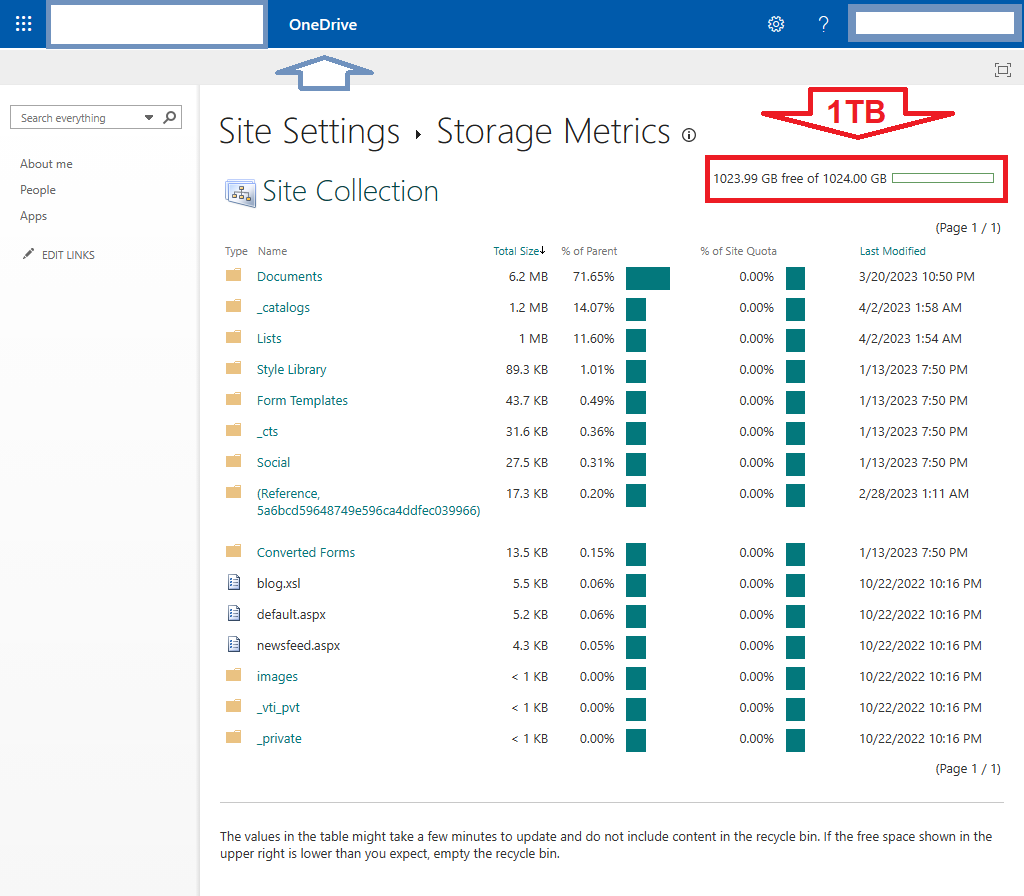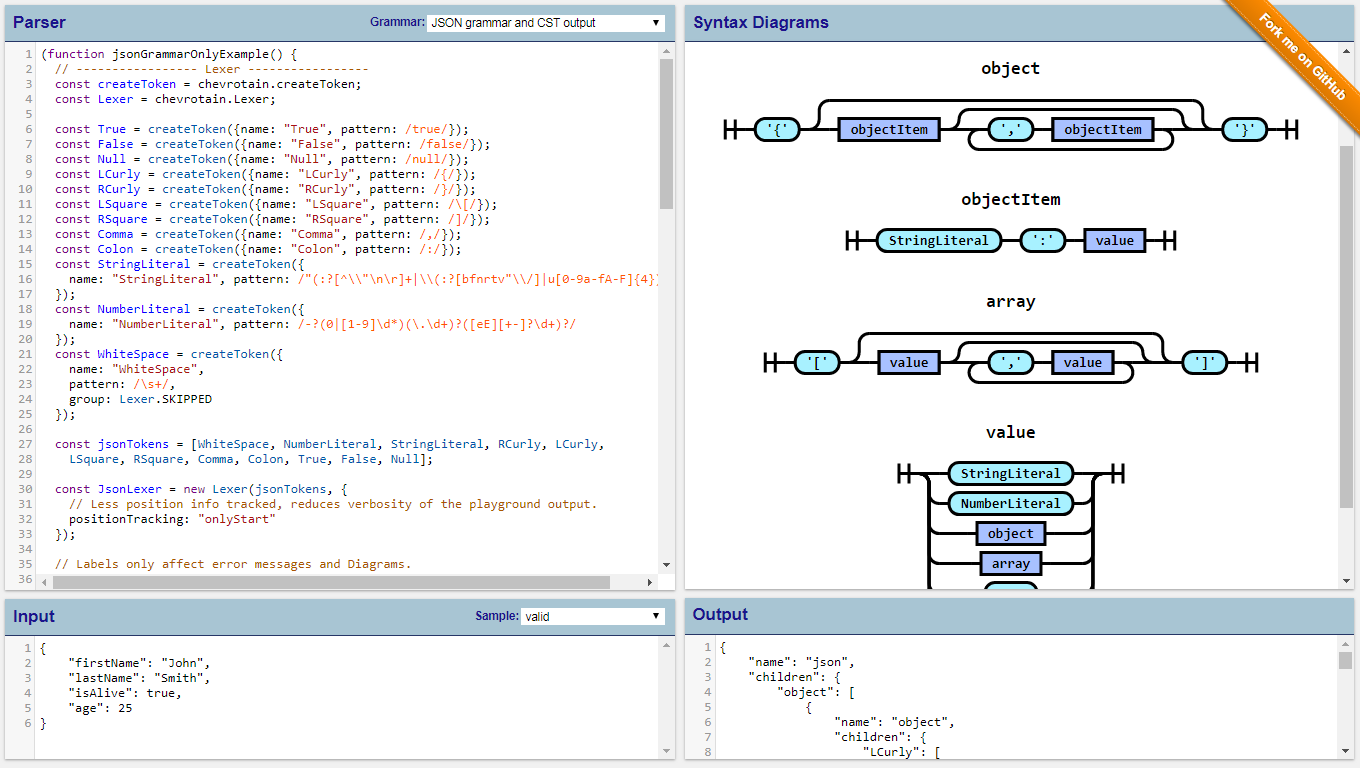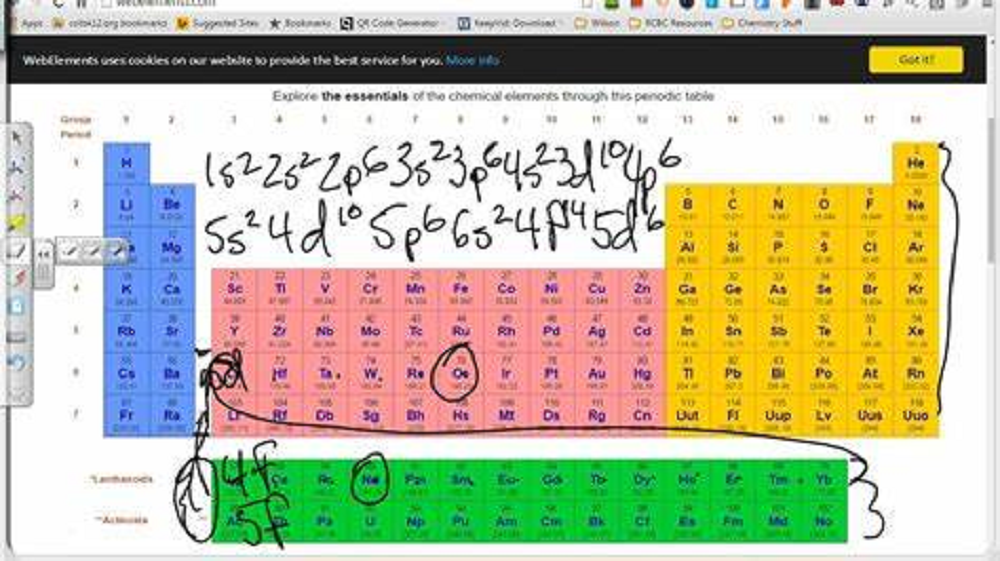### Parsering across syntax

30 + (5+13) + (7+11) + (17+19) = 30 + 18 + 18 + 36 = 30 + 36 + 36 = 102 = 2 + 100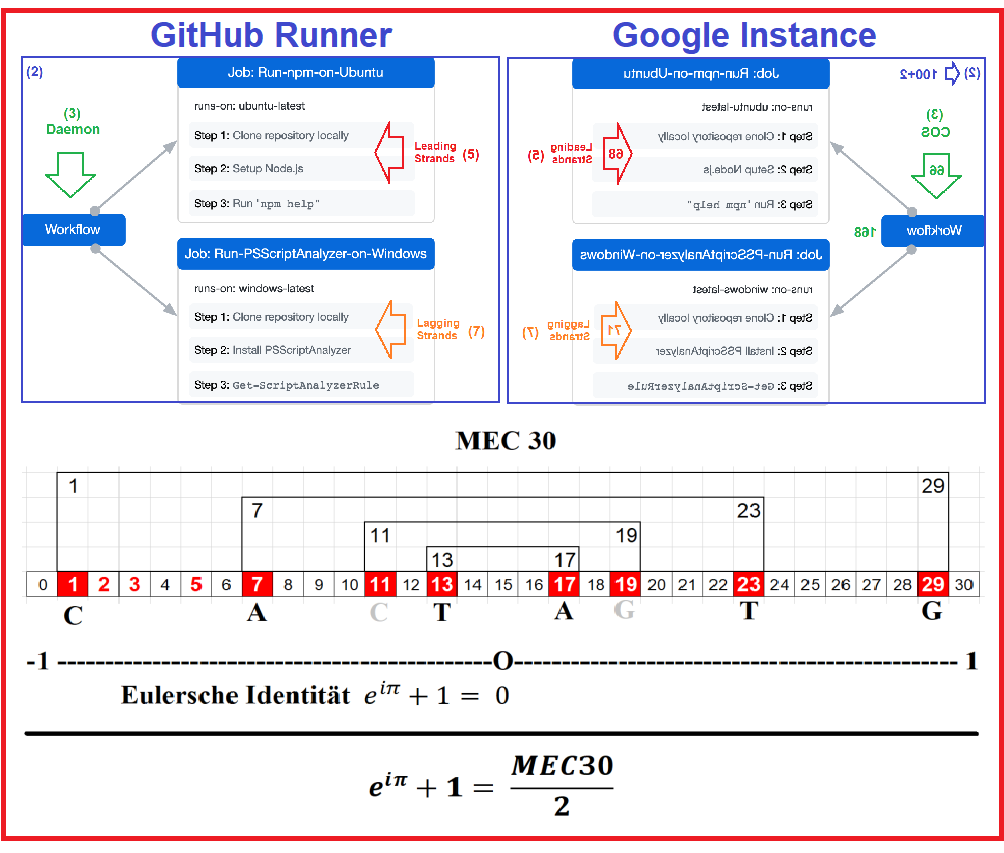### Prime images